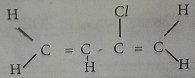# Chemistry 2020 Past Questions | WAEC

Study the following Chemistry past questions and answers for JAMBWAEC  NECO and Post-JAMB. Get prepared with official past questions and answers for upcoming examinations.

1. Which of the following substances is an example of a fine chemical?
• A. Sodium hydroxide
• B. Hydrochloric acid
• C. Ethylene
• D. Ammonia

2.  The IUPAC name of the compound represented by the structure below is ?• A. 2- chloro but-I ,3-diene.
• B. But-1,3-chlorodiene.
• C. 2-chloro but-diene.
• D. 3 -chloro but -1.3-diene.

3.  Which or the following substances is a polypeptide?

• A. Starch
• B. Glycogen
• C. Protein
• D. Fats

4.  Which or the following products could be formed during incomplete combustion of a hydrocarbon.

i.Carbon ii. Hydrogen III. Carbon (Il) oxide

• A. I only
• B. I and II only
• C. I and III only
• D. II and III only

5.

1. What quantity of electrons is lost when one mole of iron (II) ions is oxidized to iron (III) ?
• A. 0 mole
• A. 3 moles
• B. I mole
• C. 2 moles

6.  What is the mass of silver deposited when 24,125 C of electricity is passed through a solution or silver salt.

[Ag = 108, IF = 96,500 C ]

• A. 432g
• B. 108g
• C. 54g
• D. 27g

7.  Equal masses of calcium trioxocarbonate(iv) were added to dilute hydrochloric acid at the temperature specified. Under which of the following conditions would the reaction be slowest?

• A. Calcium trioxocarbonate ( iv ) chips a 20°C
• B. CalcIum trioxocarbonate (IV) chips at 40°C
• C. Calcium trioxocarbonate (IV) powder at 20’C
• D. Calcium trioxocarbonate (IV) powder at 40’C

8.  The high solubility of ethanol in water is due to?

• A. its low boiling point.
• B. its low freezing point.
• C. its covalent nature
• D. hydrogen bonding

9.  Which of the following pairs of properties of alkali metals decreases down the group?

• A. First ionization energy and reactivity
• B. melting point and atomic radius
• C. Reactivity and electronegativity
• D. First ionization energy and melting point

10.  The most suitable process of obtaining water from an aqueous solution of sugar i

• A. Crystallization
• B. Distillation
• C. Filtration
• D. Decantation

11.  Group VIl elements in their combined states are called?

• A. Halogens
• B. Anions
• C. Halide
• D. Cations

12.  When an ionic bond is broken, bonding electrons are

• A. shared between participating atoms
• B. gained by the most electropositive atom
• C. gained by the most electronegative atom
• D. lost by both participating atoms

13.  The oxidation state of chlorine in NaClO3is?

• A. +1
• B. +3
• C. +5
• D. +6

14.  A balanced chemical equation is based on the law of?

• A. Periodicity
• B. Constant composition
• C. Multiple proportion
• D. Conservation of mass

15.  Which of the following pairs of elements has the greatest difference in electronegativity?

• A. Na and F
• B. Na and CI
• C. Na and Br
• D. Na and I

## Post suggestions Here

Subscribe
Notify ofInline Feedbacks